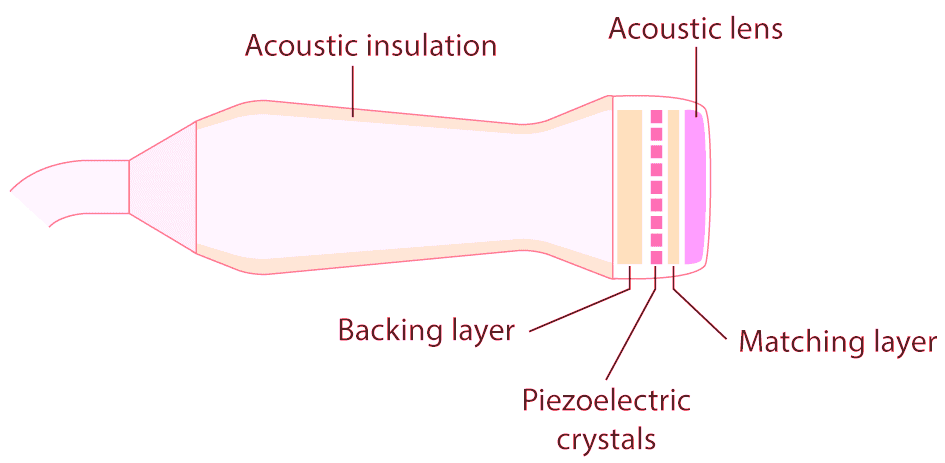# What is Piezoelectric Sensor – Construction, Working & Applications

## Piezoelectric Sensors, Construction, Working and Applications

### Piezoelectric Effect

Piezo is a Greek term signifying “press” or “squeeze”. Piezoelectricity (also called the piezoelectric effect) is the presence of an electrical potential across the sides of a crystal when mechanical stress is applied by squeezing it. In working system, the crystal acts like a tiny battery with a positive charge on one face and a negative charge on the opposite face. To make it as a complete circuit, two faces are connected together and current is passed through it.Examples:

• Mechanical stress analyzer: During construction of building, stress analyzer is used for columns, where the voltage produced due to stress is measured, and corresponding stress is calculated.
• Lighters: Gas Burner lighter and cigarette lighter utilizes piezoelectric effect. They produce electric pulse due to force developed by sudden impact of trigger over the material inside.

Inverse Piezoelectric Effect

The effect is reversible. Whenever an electric field is applied onto crystal terminals, it undergoes mechanical stress and results in shape change. This is known as Inverse Piezoelectric Effect.

Examples:

• Quartz Watch: In our day-to-day usage, the wrist watch uses quartz resonator that work as an oscillator. The element used is silicon dioxide. When an electric signal is applied across the crystal, the crystal vibrates which helps to regulate the gear inside the watch periodically.
• Piezo Buzzers: In many applications like car reverse indicator, computers, etc. buzzers are used. A certain amount of voltage with a value of magnitude and frequency is applied on the crystal, which makes the crystal to vibrate. The vibration is converted into sound.

Related Post: What is a Sensor? Different Types of Sensors with Applications

### What is a Piezoelectric Sensor?

When a sensor works on the principle of Piezoelectricity, it is known as Piezoelectric Sensor. Piezoelectricity is a phenomenon where electricity is generated if mechanical stress is applied to a material. A sensor that employs the piezoelectric effect, to measure variations in acceleration, strain, pressure, and force by converting them into electrical charge is named a piezoelectric sensor. This piezoelectricity produced is proportional to the stress given to substrates of the strong piezoelectric crystal.

### Working of Piezoelectric Sensor

When pressure or acceleration is applied to the PZT material, an equivalent amount of electrical charge gets generated across the crystal faces. Electrical charge will be proportional to the applied pressure. Piezoelectric sensor cannot be used to measure static pressure. At the constant pressure, the output signal will be zero. Working of a Piezoelectric Sensor can be summarized as,

1. In a piezoelectric crystal the charges are exactly balanced in unsymmetrical arrangement also.
2. The effect of the charges cancel out with each other and hence no net charge will be found on the crystal faces.
3. When the crystal is squeezed, the charge in the crystal becomes unbalanced.
4. Hence, from now on the effect of charge does not cancel with each other which make net positive and negative charge to appear on the opposite faces of the crystal.
5. Therefore, by squeezing the crystal, voltage is produced across the opposite face and this is known as piezoelectricity.

### Piezoelectric Sensor Circuit

The piezoelectric sensor circuit is shown below. It consists of internal Resistance Ri which is also known as insulator resistance. An inductor is connected which produces inductance due to inertia of sensor. The value of capacitance Ce is inversely proportional to the elasticity of the sensor material. To obtain the complete response of the sensor, load and leakage resistance should be larger enough such that low frequency is maintained.

### Applications of Piezoelectric Sensors:

• Piezoresistive Pressure Sensors:

They are used to measure dynamic pressure. Dynamic pressure measurements include turbulence, engine combustion, etc. The pressure changes in liquids and gases in cylinder pressure measurements, hydraulic process can be measured using piezoresistive pressure sensors.When a force is applied to the piezoelectric diaphragm, it generates electric charge across the crystal faces. The output is measured as voltage which is proportional to the applied pressure.

• Ultrasound Transducer and Piezoelectric Crystals:

Ultrasound sensors generate ultrasonic waves. When transducer is held on one hand, its position is kept varying such that the ultrasound wave passes through the body parts to be analyzed and visualized. Sound waves are sent through the body tissue. The waves are reflected back to create the image of the tissue. This is the working principle of an Ultrasound Imaging System. In this, piezoelectric crystals are attached to the front of the transducer, which helps to generate ultrasonic waves. Electrodes act as connecting node between crystals and machine. When electric signal is applied to crystal, due to vibration it generates ultrasound wave of frequencies between 1.5 and 8 MHz• In Industrial Applications piezoelectric sensor is used in engine knock sensors, pressure sensors, Sonar Equipment, etc.
• Piezoelectric actuators are applied in Diesel fuel injectors, optical adjustment, Ultrasonic cleaning and welding.
• Sensor is used in electrical appliances like dot matrix printer, inkjet printer, Piezo speaker, buzzers, humidifiers, etc.
• In musical instruments like Instrument pickups and microphone

Related Posts: